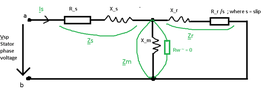# Induction motor calculation problems

• Engineering
Martin Harris
Homework Statement:
Given the 3-phased induction machine working as a motor in AC, please see the following diagram attached below, and its parameters.
Relevant Equations:
Kirchoff's Law
Ohm's Law
Given the following input parameters:
 Parameter Value Rs (Resistance through stator) 1.4 Ω Rr (Resistance through stator) 0.7 Ω Ls (stator inductance) = Lr (rotor inductance) 0.002 H xs = xr = 2*π*f*Ls 0.6283i Ω Lm(magnetic inductance) 0.01 H xm = 2*π*f*Lm 3.1415iΩ f (frequency) 50 Hz p (number of pairs of poles) 3 s (slip) 0.04 Vsl (Stator line voltage) - star configuration ; Vsp(Stator phase voltage) 380 V ; 220V zs = Rs +xs (1.4 + 0.6283i)Ω zm = xm because Rw = 0 (please see diagram attached below) 3.1415iΩ zr= Rr/s +xr (17.5 + 0.6283i)Ω

Please note that everything that has an underline below is treated as a phasor.

Following the main s (stator) branch, the m (magnetizing) and r (rotor) sub-branches are in parallel as they can be seen in the attached diagram.

a) (stator current) = ?
$$\underline {Is} =?$$
$$\underline {Is} = \frac {Vsl/sqrt(3)} {z_ab} (Eq1)$$
$$z_{ab} = z_s + z_{parallel} (Eq2)$$
$$z_s = R_s+x_s = (1.4 + 0.6283i) Ω (Eq3)$$
According to Microsoft Math Solver:
$$z_{parallel} = \frac {zm*zr} {zm+zr} =0.5389+3.0254i (Eq4)$$
Substituting Eq3 and Eq4 into Eq2, yields:
$$z_{ab} =(1.9389+3.6537i ) Ω$$
Hence substituting z_ab and Vsl/sqrt(3) into Eq1
$$\underline {Is} =\frac {380/sqrt(3)} {(1.9389+3.6537i ) Ω}$$
$$\underline {Is} =(24.8634−46.8523i) A$$
$$I_s =53.04080 A$$

b) φ =? (angle)
$$φ = arctan \frac {46.8523} {24.8634}$$
$$φ = 62.04 degrees$$

C) rotor current = ? magnetizing current =?
$$\underline {Ir} = \frac {\underline {Vparallel}} {z_{r}/s} (Eq5)$$
$$\underline {Vparallel} =\underline {Is}*z_{parallel}$$
$$\underline {Vparallel} =(24.8634−46.8523i) A * (0.5389+3.0254i)Ω$$
$$\underline {Vparallel} =155.1458+49.9730i$$
$$V_{parallel} = 162.9954 V$$

Substituting back into Eq5 yields:
$$\underline {Ir} = \frac {155.1458+49.9730i} {17.5 + 0.6283i }$$
$$\underline {Ir} = 8.95645348+2.53403773i$$
$$I_r = 9.3079 A$$

$$\underline {Im} = \frac {\underline {Vparallel}} {z_{m}} (Eq6)$$
$$\underline {Im} = \frac {155.1458+49.9730i} {3.1415i}$$
$$\underline {Im} = 15.9073−49.3858i$$
$$I_r = 51.8844 A$$

d) Stator Power = ?
$$\underline {P_s} = 3* \frac {U_{s}} {sqrt(3)} * I_{s}* cos (φ)$$
$$\underline {P_s} = 3* \frac {380V} {sqrt(3)} *53.04080 *cos (62.04 degrees)$$
$$\underline {P_s} = 16367.8966 W$$

e) Joule stator loss = ? Joule rotor loss = ?
$$L_{js} = 3*R_s* I_s^2$$
$$L_{js} = 3*1.4 Ω* 53.04080^2 A^2$$
$$L_{js} = 11815.9711 W$$

$$L_{jr} = 3*R_r* I_r^2$$
$$L_{jr} = 3*0.7 Ω* 9.3079^2 A^2$$
$$L_{jr} = 181.9377 W$$

$$L_{mech} = (1.5/100) * P_s = 24.5684 W$$
$$L_{ventilation} = (1/100) * P_s = 16.3789 W$$

f) Electromagnetic Power = ? Mechanical Power =? Assuming negligible L_Fe (Iron losses ~= 0)
$$P_{elm} = P_s - L_{js} = 16367.8966 W - 11815.9711 W$$
$$P_{elm} = 4551.9255 W$$

$$P_{mech} = P_{elm} - L_{jr} = P_{elm} * (1-s)$$
$$P_{mech} = 4551.9255 W -181.9377 W$$
$$P_{mech} = 4369.9878 W$$

g)Electromagnetic torque =? Shaft torque = ?

$$M_{electromagnetic} = \frac {P_{elm}} {Ω_s}$$
$$Ω_s = \frac {2*π*f} {p} = 104.7197 rad/s$$
$$M_{electromagnetic} = \frac {4551.9255 W} {104.7197 rad/s }$$
$$M_{electromagnetic} = 43.4677 Nm$$

$$M_{shaft} = \frac {P_{mech}} {Ω}$$
$$Ω = Ω_s*(1-s) = 100.5309 rad/s$$
$$M_{shaft} = \frac {4369.9878 W} {100.5309 rad/s }$$
$$M_{shaft} = 43.4691Nm$$

I think I am doing something wrong because Kirchoff's Law, doesen't seem to apply such that the the sum of the currents sub-branches Im+Ir > Is (main current).

Was expecting Is (main stator current) = Im+Ir (as the 2 sub-branches run in parallel)

Furthermore the Joule Stator Loss seems to be enormous Ljs =11.81 kW given that the stator Power was calculated as Ps =16.367 kW.

For sure I did something wrong, and I still didn't realize it, and that's why I need your help. I would be more than grateful if someone could check my calculations. Many thanks!

#### Attachments

•CircuitDiagram.PNG
9 KB · Views: 46
Last edited:

Gordianus
Once again, I'm reading the Forum with my phone and my eyes aren't what they used to be. I'll check later with a large PC screen. Lm is too small

•Martin Harris
Martin Harris
Once again, I'm reading the Forum with my phone and my eyes aren't what they used to be. I'll check later with a large PC screen. Lm is too small
Right, okay. Thanks a lot!
That was the given Lm = 0.01 H

I just find it weird that Kirchoff's Law, doesen't seem to apply such that the the sum of the currents sub-branches Im+Ir > Is (main current).

Furthermore the Joule Stator Loss seems to be enormous Ljs =11.81 kW given that the stator Power was calculated as Ps =16.367 kW.

Gordianus
I checked your calculations and, at first glance, found no mistakes.
I insist, Lm is too small. This motor has no core!
No wonder the stator current is huge and the power losses shoot through the roof.
Let's assume the motor has no load (s=0). If you repeat the calculation will find the stator current is still too large. This is crazy. A good motor with no load should have a low stator current.

•Martin Harris
••